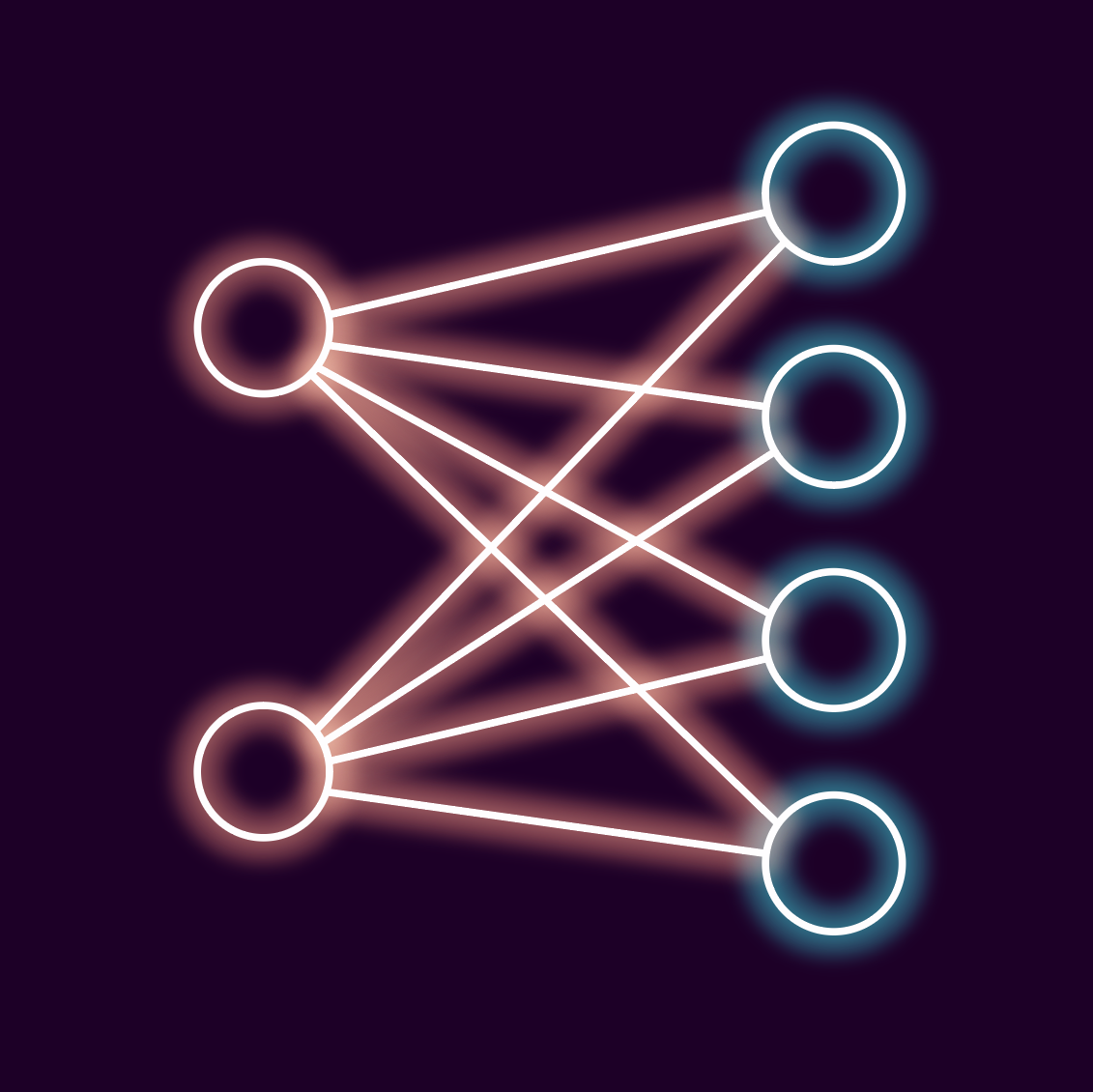# 🧠 自己用 JavaScript 手寫：人工智慧/神經網路......！ 😱 不使用任何套件！🤯

## 第一步

1. X > 0 且 Y > 0

2. X < 0 且 Y > 0

3. X > 0 且 Y < 0

4. X < 0 且 Y < 0### 我們的第一個神經元

``````class NeuralNetwork {
constructor(inputLen, outputLen) {
this.inputLen = inputLen;
this.outputLen = outputLen;
this.weights = Array.from({ length: this.outputLen }, () =>
Array.from({ length: this.inputLen }, () => Math.random())
);
this.bias = Array(this.outputLen).fill(0);
}
}

const neuralNetwork = new NeuralNetwork(2, 4);
``````

`this.inputLen = inputLen;``this.outputLen = outputLen;` 只是為了讓我們可以引用輸入和輸出的數量。

`this.weights = [...]` 是連線。現在看起來可能有點嚇人，但這就是我們正在做的事情：

• 建立輸出神經元陣列（`outputLen`

• 將長度為“inputLen”的陣列加入到每個陣列條目，並用 0 到 1 之間的一些隨機值填充它以開始我們的工作。

``````this.weights = [
[0.7583747881712366,0.4306037998314902],
[0.40553698492617807,0.4419651593960727],
[0.852978801662627,0.9762509253699836],
[0.8701610553353811,0.5583309725764114]
]
``````

``````[input 1 to output 1, input 2 to output 1],
[input 1 to output 2, input 2 to output 2],
[input 1 to output 3, input 2 to output 3],
[input 1 to output 4, input 2 to output 4],``````

### 使用我們的網路！

``````propagate(inputs) {
const output = new Array(this.outputLen);
for (let i = 0; i < this.outputLen; i++) {
output[i] = 0;
for (let j = 0; j < this.inputLen; j++) {
output[i] += this.weights[i][j] * inputs[j];
}
output[i] += this.bias[i];
output[i] = this.sigmoid(output[i]);
}
return output;
}

sigmoid(x) {
return 1 / (1 + Math.exp(-x));
}
``````

#### 乙狀結腸### 訓練時間到了！

``````    train(inputs, target) {
const output = this.propagate(inputs);
const errors = new Array(this.outputLen);

for (let i = 0; i < this.outputLen; i++) {
errors[i] = target[i] - output[i];
for (let j = 0; j < this.inputLen; j++) {
this.weights[i][j] +=
this.learningRate *
errors[i] *
output[i] *
(1 - output[i]) *
inputs[j];
}
this.bias[i] += this.learningRate * errors[i];
}
}
``````

“為什麼花了這麼長時間？”我聽到你問了！好吧，它讓我思考了所有需要相乘才能更新每個權重的位元。

#### 訓練資料調整

``````const trainingData = [
{ x: -0.5, y: -0.5, label: "blue" },
{ x: 0.5, y: -0.5, label: "red" },
{ x: -0.5, y: 0.5, label: "green" },
{ x: 0.5, y: 0.5, label: "purple" }
];``````

## 我們有一個有效且有用的神經網路！

``````function train() {
for (let i = 0; i < 10000; i++) {
const data =
trainingData[Math.floor(Math.random() * trainingData.length)];
neuralNetwork.train([data.x, data.y], encode(data.label));
}
console.log("Training complete");
}
``````

### 金髮女孩迭代

10,000 次迭代足以訓練這個特定的神經網路。但對於我們稍後將介紹的更複雜的問題，我需要更多的迭代（並降低學習率）。

## 簡單的普通 JS 神經網路演示

「encode」和「decode」純粹是為了獲取我們的輸出神經元，找到哪個神經元具有最高的激活，然後將其映射到我們可視化的顏色。

``````// this line finds the max value of all of our output neurons and then returns its index so we can use that to classify our X and Y coordinates.
const maxIndex = output.indexOf(Math.max(...output));``````

### 用法和實際演示

• 訓練 - 在我們的神經網路開始未經訓練和隨機化時對其進行訓練。

• 分類點 - 這是為了執行我們的神經網路。它將在圖表上繪製點並為它們分配顏色。我建議在訓練之前和之後執行這個。

• 重設 - 這將建立一個未經訓練的新神經網路。非常適合在訓練前後測試點的分類。

https://codepen.io/GrahamTheDev/pen/abPxoqb

## 版本 2 - 隱藏神經元### 那麼有什麼新內容呢？

``````class NeuralNetwork {
constructor(inputSize, hiddenSize, outputSize) {
this.inputSize = inputSize;
this.hiddenSize = hiddenSize;
this.outputSize = outputSize;
this.weightsInputToHidden = Array.from({ length: hiddenSize }, () =>
Array.from({ length: inputSize }, () => Math.random() * 2 - 1)
);
this.biasHidden = Array(hiddenSize).fill(0);
this.weightsHiddenToOutput = Array.from({ length: outputSize }, () =>
Array.from({ length: hiddenSize }, () => Math.random() * 2 - 1)
);
this.biasOutput = Array(outputSize).fill(0);
this.learningRate = document.querySelector('#learningRate').value; // Adjusted learning rate
this.hiddenLayer = new Array(this.hiddenSize);
}

feedForward(inputs) {
for (let i = 0; i < this.hiddenSize; i++) {
this.hiddenLayer[i] = 0;
for (let j = 0; j < this.inputSize; j++) {
this.hiddenLayer[i] +=
this.weightsInputToHidden[i][j] * inputs[j];
}
this.hiddenLayer[i] += this.biasHidden[i];
this.hiddenLayer[i] = sigmoid(this.hiddenLayer[i]);
}

const output = new Array(this.outputSize);
for (let i = 0; i < this.outputSize; i++) {
output[i] = 0;
for (let j = 0; j < this.hiddenSize; j++) {
output[i] +=
this.weightsHiddenToOutput[i][j] * this.hiddenLayer[j];
}
output[i] += this.biasOutput[i];
output[i] = sigmoid(output[i]);
}
return output;
}

train(inputs, target) {
for (let i = 0; i < this.hiddenSize; i++) {
this.hiddenLayer[i] = 0;
for (let j = 0; j < this.inputSize; j++) {
this.hiddenLayer[i] +=
this.weightsInputToHidden[i][j] * inputs[j];
}
this.hiddenLayer[i] += this.biasHidden[i];
this.hiddenLayer[i] = sigmoid(this.hiddenLayer[i]);
}

const output = new Array(this.outputSize);
for (let i = 0; i < this.outputSize; i++) {
output[i] = 0;
for (let j = 0; j < this.hiddenSize; j++) {
output[i] += this.weightsHiddenToOutput[i][j] * this.hiddenLayer[j];
}
output[i] += this.biasOutput[i];
output[i] = sigmoid(output[i]);
}

const errorsOutput = new Array(this.outputSize);
const errorsHidden = new Array(this.hiddenSize);

for (let i = 0; i < this.outputSize; i++) {
errorsOutput[i] = target[i] - output[i];
for (let j = 0; j < this.hiddenSize; j++) {
this.weightsHiddenToOutput[i][j] +=
this.learningRate *
errorsOutput[i] *
output[i] *
(1 - output[i]) *
this.hiddenLayer[j];
}
this.biasOutput[i] += this.learningRate * errorsOutput[i];
}

for (let i = 0; i < this.hiddenSize; i++) {
errorsHidden[i] = 0;
for (let j = 0; j < this.outputSize; j++) {
errorsHidden[i] += this.weightsHiddenToOutput[j][i] * errorsOutput[j];
}
this.biasHidden[i] += this.learningRate * errorsHidden[i];
for (let j = 0; j < this.inputSize; j++) {
this.weightsInputToHidden[i][j] +=
this.learningRate *
errorsHidden[i] *
this.hiddenLayer[i] *
(1 - this.hiddenLayer[i]) *
inputs[j];
}
}
}
}
``````

### 加入一些變數來玩

• 訓練資料大小 - 我們產生的不同隨機點的數量

• 訓練迭代 - 我們從訓練集中選擇隨機資料點並將其輸入神經網路上的「訓練」函數的次數。

• 學習率 - 我們根據錯誤調整速度的乘數。

• 隱藏節點（超過2個！） - 調整第二層有多少個隱藏節點（需要您再次初始化網絡，否則會損壞！）

• 要分類的點 - 傳遞給我們訓練過的神經網路並繪製在圖表上的點數。

## 演示

https://codepen.io/GrahamTheDev/pen/qBLwBxP